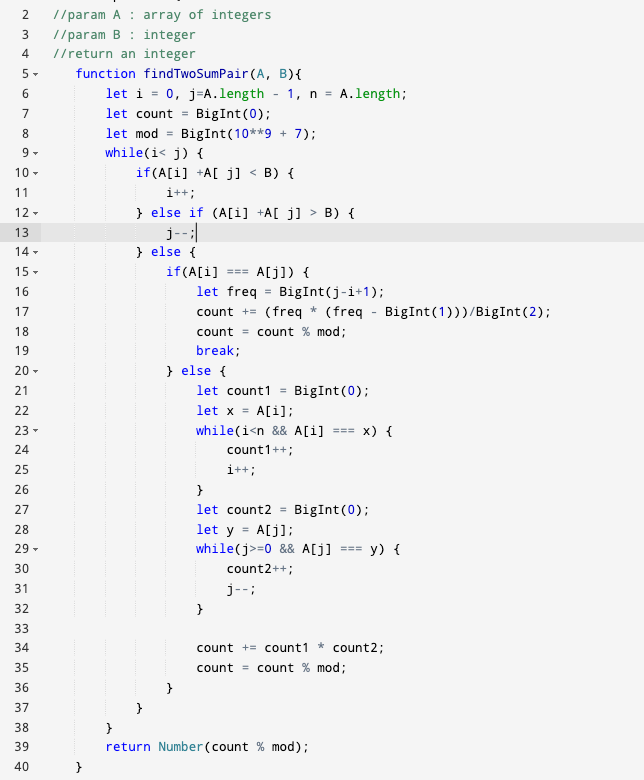top of page

# Title: Mastering the Art of Two Pointers: Unraveling the Secrets to Solve Complex Problems

Solving problems using two-pointers(not c/c++ pointers) is like orchestrating a symphony; it requires precision, finesse, and a deep understanding of the melody. In this article, we will explore the intricacies of two pointers through two classic problems: finding pairs with a sum in a sorted array and checking if a string is a palindrome. By unraveling the underlying principles and sharing essential tips, we aim to empower you to tackle similar challenges with confidence and flair. Q.1 Finding Pairs with Sum B in a Sorted Array:

Problem Statement:

Given a sorted array of integers (not necessarily distinct) A and an integer B, find and return how many pairs of integers (A[i], A[j]) such that i != j have a sum equal to B.

Observations:

• Initial Placement of Pointers:

• Place one pointer at the beginning of the array (left = 0) and another at the end (right = len(A) - 1).

• Moving the Pointers:

• Move the left pointer forward if A[left] + A[right] < B. Since the array is sorted, increasing the left pointer ensures a larger sum.

• Move the right pointer backward if A[left] + A[right] > B. Moving the right pointer decreases the sum.

Code in Javascript:Insights:

• The two-pointer approach reduces the time complexity to O(N) compared to O(N^2) of the brute force method.

• The pointers efficiently converge towards the target sum, ensuring an optimal solution.

Conclusion:

Mastering the art of two-pointers is not just about algorithms; it's about intuition, strategy, and problem-solving finesse. By understanding where to place your pointers and how to move them smartly, you can elegantly navigate through a myriad of problems, as showcased in the examples above. Armed with this knowledge, you are equipped to unravel the complexities of various problems, creating extraordinary solutions that captivate both the mind and the code.

So, dive in, experiment, and let the symphony of two-pointers guide you to unparalleled problem-solving mastery!